# Texas Go Math Grade 4 Lesson 12.3 Answer Key Model Perimeter Formulas

Refer to our Texas Go Math Grade 4 Answer Key Pdf to score good marks in the exams. Test yourself by practicing the problems from Texas Go Math Grade 4 Lesson 12.3 Answer Key Model Perimeter Formulas.

## Texas Go Math Grade 4 Lesson 12.3 Answer Key Model Perimeter Formulas

Essential Question

How can you use a formula to find the perimeter of a rectangle?
Perimeter of rectangle = (2 × length) + (2 × width)
Rectangle consists of four sides and the sum of all length of sides is the perimeter of rectangle.
Explanation: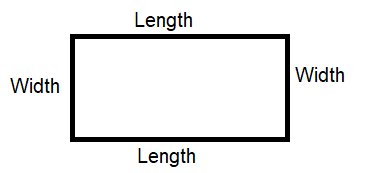Perimeter of a Rectangle = length + width + length + width

Unlock the Problem

Julio is putting a stone border around his rectangular garden. The length of the garden is 7 feet. The width of the garden is 5 feet. How many feet of stone border does Julio need?

• Circle the numbers you will use.
• What are you asked to find?

24 feet
Explanation:
The length of the garden is 7 feet. The width of the garden is 5 feet
7 + 5 + 7 + 5 = 24
The perimeter is 24 feet.
So, Julio needs 24 feet of stone border.

Perimeter is the distance around a figure.

To find how many feet of stone border Julio needs, find the perimeter of the garden.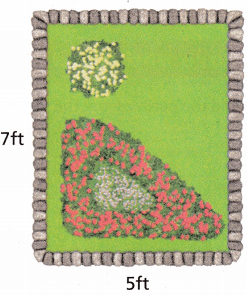Perimeter of a Rectangle = length + width + length + width
7 + 5 + 7 + 5 = __________
The perimeter is __________ feet.
So, Julio needs __________ feet of stone border.
Explanation:
Perimeter of a Rectangle = length + width + length + width
7 + 5 + 7 + 5 = 24
The perimeter is 24 feet.
So, Julio needs 24 feet of stone border.

Use Multiplication

(A) Find Perimeter of a rectangle
Perimeter = (2 × length) + (2 × width), or 2l + 2wPerimeter = (2 × 12) + (2 × 8)
= 24 + 16
= ____________
So, the perimeter is __________ centimeters.
Explanation:
length = 12 cm
width = 8 cm
Perimeter = (2 × 12) + (2 × 8)
= 24 + 16 = 40 centimeter
So, the perimeter is 40 centimeters.

(B) Find Perimeter of a Square
Perimeter = 4 × one side, or 4 × sPerimeter = 4 × 16
= ___________
So, the perimeter is ___________ inches.
Explanation:
Perimeter = 4 × 16 = 64 inches
So, the perimeter is 64 inches.

Use a Formula A formula ¡s a mathematical rule. You can use a formula to find perimeter.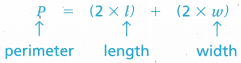Example Find the perimeter of the rectangle.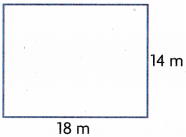P = (2 × l) + (2 × w)
= (2 × ______ ) + (2 × __________ ) Think: Write the measures you know.
= __________ + ___________ Think: Do what is in parentheses first.
= ___________
The perimeter of the rectangle is ______________ .
P = (2 × l) + (2 × w)
= (2 × 18) + (2 × 14) Think: Write the measures you know.
= 36 + 28 Think: Do what is in parentheses first.
= 64 m
The perimeter of the rectangle is 64 m.
Explanation:
A rectangle is a parallelogram all of whose angles are right angles; especially one with adjacent sides of unequal length.
It has 4 sides and 4 vertices
Perimeter of rectangle = (2 × length) + (2 × width)

Share and Show

Question 1.
Find the perimeter of the rectangle.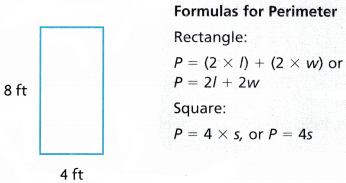P = (___________ × ___________) + (___________ × ___________)
= (___________ × ___________) + (___________ × ___________)
= ___________ + ___________
= ___________
The perimeter is ___________ feet.
P = (2 × l) + (2 × w)
P = (2 x 8) + (2 x 4)
= 16 + 8 =  24 ft
Explanation:
A rectangle is a parallelogram all of whose angles are right angles; especially one with adjacent sides of unequal length.
It has 4 sides and 4 vertices.
Perimeter of rectangle = (2 × length) + (2 × width)

Find the perimeter of the rectangle or square.

Question 2.___________ yards
40 yards
Explanation:
Perimeter of rectangle (p) = (2 × length) + (2 × width)
P = (2 × l) + (2 × w)
P = (2 x 16) + (2 x 4)
P = 40 yards

Question 3._____________ meters
Explanation:
Perimeter of rectangle (p) = (2 × length) + (2 × width)
P = (2 × l) + (2 × w)
P = (2 x 42) + (2 x 110)
= 84 + 220
= 304 m

Question 4._____________ meters
Explanation:
Perimeter of square = 4 x side
P = 4 x 4 = 16 m

Math Talk

Mathematical Processes
Can you use the formula P = (2 × l) + (2 × w) to find the perimeter of a square? Explain.
Explanation:
Perimeter ‘P’
Perimeter of rectangle is P
Perimeter of a Rectangle = length + width + length + width
P = (2 × length) + (2 × width)Unlock the Problem

Question 5.H.O.T. Multi-step Alejandra plans to sew fringe on a scarf. The scarf is shaped like a rectangle. The length of the scarf is 48 inches. The width is one half the length. How much fringe does Alejandra need?
(A) 72 inches
(B) 96 inches
(C) 120 inches
(D) 144 inches
Option(D)
Perimeter of a Rectangle = length + width + length + width
P = (2 × length) + (2 × width)
=  (2 x 48) + (2 x 24)
=  96 + 48  = 144 inches

a. Draw a picture of the scarf, and label the given measurements on your drawing.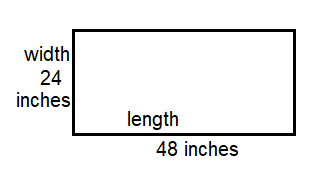b. What do you need to find?
The perimeter of the scarf
c. What formula will you use?
Perimeter of rectangle.
P = (2 × length) + (2 × width)

d. Show the steps you use to solve the problem.
Perimeter of a Rectangle = length + width + length + width
P = (2 × length) + (2 × width)
=  (2 x 48) + (2 x 24)
=  96 + 48
= 144 inches

e. Complete.
‘The length of the scarf is __________ inches.
The width is one half the length or __________ ÷ 2.
The width is __________ inches.
So, the perimeter is (__________ × __________) + ( __________ × __________) = __________ inches.
Fill in the bubble for the correct answer choice above.
‘The length of the scarf is 48 inches.
The width is one half the length or 48 ÷ 2.
The width is 24 inches.
So, the perimeter is ( 2 × 48) + (2 ×24) = 144 inches.

Question 6.
H.O.T. Reasoning What is the side length of a square with a perimeter of 44 centimeters?
(A) 4 centimeters
(B) 11 centimeters
(C) 22 centimeters
(D) 176 centimeters
Option(D)
Explanation
P = 4 x side
= 4 x 44
= 176 centimeters

Question 7.
Apply Mr. Wong is putting a brick edge around his rectangular patio. What is the perimeter of the patio?(A) 28 ft
(B) 38 ft
(C) 56 ft
(D) 66 ft
Option(C)
Explanation:
Perimeter of a Rectangle = length + width + length + width
P = (2 × length) + (2 × width)
=  (2 x 18) + (2 x 10)
= 36 + 20
= 56 ft

Question 8.
A rectangular swimming pool is 24 feet long and 12 feet wide. What is the perimeter of the swimming pool?
(A) 288ft
(B) 36ft
(C) 60ft
(D) 72ft
Option(D)
Explanation:
Perimeter of a Rectangle = length + width + length + width
P = (2 × length) + (2 × width)
=  (2 x 24) + (2 x 12)
= 48 + 24
=  72 ft

Question 9.
Todd is putting a fence around a square shaped section of his backyard. The square section is 102 m on each side. How many meters of fence does Todd need in all?(A) 408 m
(B) 104 m
(C) 800 m
(D) 204 m
Option(A)
Explanation:
Perimeter of a Rectangle = length + width + length + width
P = 4 × side
=  (4 x 102)
= 408 m

Question 10.
Multi-Step Joe is putting payers around his rectangular patio. The width of the patio is 14 feet. The length is three times the width. flow many feet of payers does he need?
(A) 34 ft
(B) 588 ft
(C) 112 ft
(D) 168 ft
Option (C)
Explanation:
Perimeter of a Rectangle = length + width + length + width
P = (2 × length) + (2 × width)
=  (2 x 14) + (2 x 3×14)
= 28 + 84 = 112

TEXAS Test Prep

Question 11.
Lola is putting a border on the quilt she is making. The quilt is shaped like a rectangle. The width of the quilt is 48 inches and its length is twice its width. How much border does Lola need?
(A) 96 inches
(B) 288 inches
(C) 144 inches
(D) 192 inches
Option(B)
Explanation:
Perimeter of a Rectangle = length + width + length + width
P = (2 × length) + (2 × width)
=  (2 x 48) + (2 x 2 x 48)
=  96 + 192 = 288 inches

### Texas Go Math Grade 4 Lesson 12.3 Homework and Practice Answer Key

Find the perimeter of the rectangle or square.

Question 1.____________ feet
Explanation:
Perimeter of a Rectangle = length + width + length + width
P = (2 × length) + (2 × width)
=  (2 x 6) + (2 x 8)
= 12 + 16 = 28 ft

Question 2.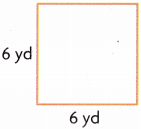____________ yards
24 yards
Explanation:
P = 4 x side
= 4 x 6 = 24 yd

Question 3.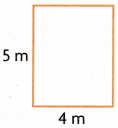____________ meters
18 meters
Explanation:
Perimeter of a Rectangle = length + width + length + width
P = (2 × length) + (2 × width)
=  (2 x 5) + (2 x 4)
= 10 + 8 = 18 meters

Question 4.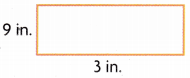____________ inches
24 inches
Explanation:
Perimeter of a Rectangle = length + width + length + width
P = (2 × length) + (2 × width)
=  (2 x 9) + (2 x 3) =18 + 6 = 24 in

Question 5.____________ yards
320 yards
Explanation:
P = 4 x side
= 4 x 80 = 320 yd

Question 6.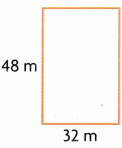____________ meters
Explanation:
Perimeter of a Rectangle = length + width + length + width
P = (2 × length) + (2 × width)
=  (2 x 48) + (2 x 32)
= 96 + 64 = 160 m

Problem Solving

Question 7.
Mrs. Sanders will put a border around a bulletin board that is 52 inches long and 30 inches wide. How many inches of border will she need?
164 inches
Explanation:
Perimeter of a Rectangle = length + width + length + width
P = (2 × length) + (2 × width)
=  (2 x 52) + (2 x 30)
= 104 + 60 = 164 inches

Question 8.
A rectangular room is 10 meters wide and 14 meters long. What is its perimeter?
48 meters
Explanation:
Perimeter of a Rectangle = length + width + length + width
P = (2 × length) + (2 × width)
=  (2 x 10) + (2 x 14) = 20 + 28 = 48 meters

Lesson Check

Question 9.
What is the side length of a square with a perimeter of 60 meters?
(A) 240 meters
(B) 30 meters
(C) 4 meters
(D) 15 meters
Option (A)
Explanation:
Perimeter of a Rectangle = length + width + length + width
P = (2 × length) + (2 × width)
=  4 x 60 = 240 meters

Question 10.
Robert wants to put lights around the edge of his yard. The yard is 40 feet long and 25 feet wide. How many feet of lights does he need?
(A) 1000 feet
(B) 130 feet
(C) 100 feet
(D) 160feet
Option(B)
Explanation:
Perimeter of a Rectangle = length + width + length + width
P = (2 × length) + (2 × width)
=  (2 x 40) + (2 x 25) = 80 + 50 = 130 feet

Question 11.
Each side of a table is 3 meters long. What is the perimeter of the table?
(A) 30 meters
(B) 9 meters
(C) 6 meters
(D) 12 meters
Option(D)
Explanation:
Perimeter P = 4 x side
= 4 x 3 = 12 meters

Question 12.
The movie screen at the cinema is 70 feet long and 30 feet wide. What is the perimeter of the screen?
(A) 200 feet
(B) 140 feet
(C) 60 feet
(D) 2100 feet
Option (A)
Explanation:
Perimeter of a Rectangle = length + width + length + width
P = (2 × length) + (2 × width)
=  (2 x 70) + (2 x 30) =140 + 60 = 200 feet

Question 13.
Multi-Step Marcia will make a frame for her picture. The length of the picture is 15 inches. The width is one third of the length. How much wood does Marcia need for the frame?
(A) 18 inches
(B) 40 inches
(C) 30 inches
(D) 45 inches
Option(B)
Explanation:
Perimeter of a Rectangle = length + width + length + width
P = (2 × length) + (2 × width)
=  (2 x 15) + (2 x 5) = 30 + 10 = 40 inches

Question 14.
Multi-Step Greg will build a fence for his yard. The width of the yard is 12 feet. The length of the yard is twice its width. How much fencing will Greg need for his yard?
(A) 60 feet
(B) 48 feet
(C) 24 feet
(D) 72 feet